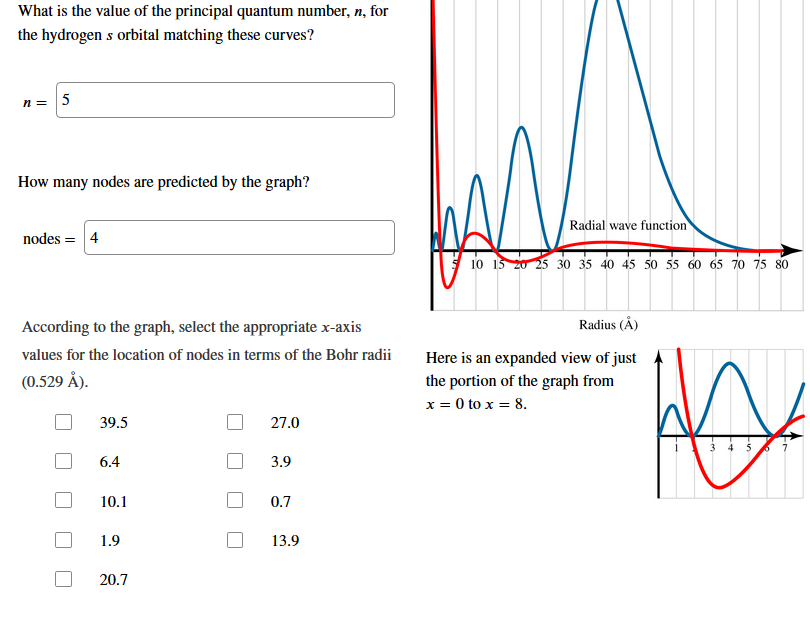# What is the value of the principal quantum number, n, forthe hydrogen s orbital matching these curves?n=How many nodes are predicted by the graph?Radial wave functionnodes410 15 0 2530 35 40 45 50 55 60 65 70 75 80Radius (A)According to the graph, select the appropriate x-axisvalues for the location of nodes in terms of the Bohr radiiHere is an expanded view of just(0.529 Å)the portion of the graph fromx 0 to x 839.527.03 4 56.43.910.10.71.913.920.7

Question
617 viewshelp_outlineImage TranscriptioncloseWhat is the value of the principal quantum number, n, for the hydrogen s orbital matching these curves? n= How many nodes are predicted by the graph? Radial wave function nodes 4 10 15 0 2530 35 40 45 50 55 60 65 70 75 80 Radius (A) According to the graph, select the appropriate x-axis values for the location of nodes in terms of the Bohr radii Here is an expanded view of just (0.529 Å) the portion of the graph from x 0 to x 8 39.5 27.0 3 4 5 6.4 3.9 10.1 0.7 1.9 13.9 20.7 fullscreen
check_circle

Step 1

(1) The principal quantum number can be given by the below mentioned formula:

Principal quantum number(n) = Number of nodes+1

As per the given curve, there are 4 nodes

Substitute the same in the above formula,

Principal quantum number(n) = 4 + 1 = 5

2) There are 4 nodes located.

Step 2

3) The radial wave function curve is represented by red in the image given. The values for the location of nodes on the X−axis is to be selected by choosing the points, where the curve cuts the X−axis. The curve cuts the X-axis at 4 different points which are 1.9, 6.4,13.9 and 27 (most appropriate from the given list)

Step 3

### Want to see the full answer?

See Solution

#### Want to see this answer and more?

Solutions are written by subject experts who are available 24/7. Questions are typically answered within 1 hour.*

See Solution
*Response times may vary by subject and question.
Tagged in
ScienceChemistry

### Atomic structure